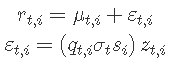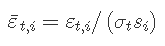# R语言乘法GARCH模型对高频交易数据进行波动性预测

getSymbols('C')
R_d = ROC(Cl(C), na.pad = FALSE)

acf(abs((R_i))

## 模型## 估算

# 定义一个日模型
spec(list(armaOrder = c(1, 1)))
# 使用ugarchroll方法创建一个滚动的预测
roll(spec)
#提取sigma 预测
sigma = as.xts(df$, 'Sigma'$)
#现在估计日内模型
spec( list(model = 'mcsGARCH'))
# DailyVar是预测日方差的必要xts对象
fit(data = R, spec, Var = sigma^2)

plot([email protected]^0.5))

# R语言GARCH-DCC模型和DCC（MVT）建模估计

## 预测

forecast(fit2, n.ahead = 10, n.roll = 299, f_sigma^2)

## 仿真

# dtime包含了数据集中唯一的区间点的集合
# (可从mcsGARCH模型的所有rugarch对象中获得)
sim = sim(fit, n.sim = 10000,Var = var_sim)
#
plot(as.numeric([email protected]$DiurnalVar^0.5), type = 'l') ## 滚动的回测和风险值 ugarchroll函数对于在回测应用中测试模型的充分性非常有用，下面的代码说明了模型在数据期间的情况。 n = length(index(R_d$'2008-01-01/2008-03-01'$)) roll(spec, data = R, refit.window = 'moving') 效果比较理想。 # 如下图所示，VaR能很好地处理日内数据。观察到的VaR峰值是开盘前后的季节性因素造成的。 D = as.POSIXct(rownames([email protected]$VaR))
VaRplot(0.01, actual = xts([email protected]$VaR$, 3$, D), VaR = xts([email protected]$VaR$,1$, D))

## 参考文献

Bollerslev, T., & Ghysels, E. (1996). Periodic autoregressive conditional heteroscedasticity. Journal of Business & Economic Statistics, 14(2), 139–151.

Andersen, T. G., & Bollerslev, T. (1997). Intraday periodicity and volatility persistence in financial markets. Journal of Empirical Finance, 4(2), 115–158.

Kaizong Ye拓端研究室（TRL）的研究员。

​非常感谢您阅读本文，如需帮助请联系我们！QQ在线咨询

15121130882

0571-63341498

## 关注有关新文章的微信公众号

This will close in 0 seconds